Li, H., Zhang, H., Li, J., and Du, F. (2014). "Comparison of interfiber bonding ability of different poplar P-RC alkaline peroxide mechanical pulp (APMP) fiber fractions," BioRes. 9(4), 6019-6027.

#### Abstract

In recent years, the alkaline peroxide mechanical pulping performed with refiner-chemical preconditioning (P-RC APMP) has obtained a wide application in many paper grades in China. This is due to such desirable pulp characteristics as its high bulk, opacity, and light scattering coefficient. However, compared with its bleached chemical pulps, the poplar P-RC APMP has weak bonding strength, which limits its application in value-added products. Usually, the interfiber bonding abilities of different fiber fractions are quite different. In this study, the page bonding strength index (B) and the interfiber bonding abilities (shear bond strength per unit area (b) and relative bonding area (RBA)) of different P-RC APMP fiber fractions were extrapolated by the traditional Page Equation. The results show that the higher B could be obtained when the fiber fraction was shorter or smaller. The same trend was observed for b and RBA. Wet pressing was used to improve the bonding ability, and higher pressure of wet pressing or shorter fiber fraction can lead to obviously higher tensile strength.

#### Full Article

Comparison of Interfiber Bonding Ability of Different Poplar P-RC Alkaline Peroxide Mechanical Pulp (APMP) Fiber Fractions

Hailong Li, Hongjie Zhang,* Jianguo Li, and Fang Du

In recent years, the alkaline peroxide mechanical pulping performed with refiner-chemical preconditioning (P-RC APMP) has obtained a wide application in many paper grades in China. This is due to such desirable pulp characteristics as its high bulk, opacity, and light scattering coefficient. However, compared with its bleached chemical pulps, the poplar P-RC APMP has weak bonding strength, which limits its application in value-added products. Usually, the interfiber bonding abilities of different fiber fractions are quite different. In this study, the page bonding strength index (B) and the interfiber bonding abilities (shear bond strength per unit area (b) and relative bonding area (RBA)) of different P-RC APMP fiber fractions were extrapolated by the traditional Page Equation. The results show that the higher B could be obtained when the fiber fraction was shorter or smaller. The same trend was observed for b and RBA. Wet pressing was used to improve the bonding ability, and higher pressure of wet pressing or shorter fiber fraction can lead to obviously higher tensile strength.

Keywords: Poplar P-RC APMP; Fiber fractions; Interfiber bonding; Relative bonded area (RBA)

Contact information: Tianjin Key Laboratory of Pulp and Paper, Tianjin University of Science and Technology, Tianjin 300457, China; *Corresponding author: hongjiezhang@tust.edu.cn

INTRODUCTION

In recent years, alkaline peroxide mechanical pulping carried out with refiner-chemical preconditioning (P-RC APMP) has achieved widespread application in many paper grades in China (Xu 1999). Compared with the corresponding bleached chemical pulps, the poplar P-RC APMP pulps have high bulk, opacity, and light scattering coefficient, but the main drawback is its weak interfiber bonding strength, which limits its application in value-added products. Like other high-yield pulps (HYPs), P-RC APMP has various fiber fractions that not only play an important role in the papermaking process but also affect the paper properties. The differing nature of pulp fibers by their varying dimensional fractions has given rise to the variability in the fiber bonding property (Lei et al. 2013). Therefore, it is important to clarify the contributions of different P-RC APMP fiber fractions on interfiber bonding in the fiber matrix of paper sheets and subsequently improve the bonding strength of HYP-containing paper sheets.

There are many theories that express the relationship between strength properties of paper and fiber characteristics; the most widely used is the Page Equation (Page 1969). Page showed that the tensile strength of paper could be expressed in a simple and effective way by Eq. 1,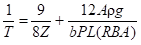(1)

where A is the average fiber cross section, ρ is the fiber density, and gis acceleration due to gravity. The product of the Aρ, and g is closely related to the fiber coarseness (c), so the Page Equation can be changed into Eq. 2: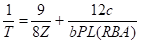(2)

The parameters T and Z in Eq. 2 are the long-span and zero-span tensile indices, respectively. The variables in Eq. 2, including fiber coarseness (c), fiber perimeter (P), and fiber length (L), are measurable fiber properties. However, the shear bond strength per unit area (b) and relative bond area (RBA) are properties of the paper sheet, not of the fibers, and are considerably more difficult to determine experimentally. The shear bond strength per unit area (b, N/m2 or MPa) is a parameter to represent the interfiber bonding ability in unit area of a paper sheet, and the differences for bparameter of paper sheets made from different fibers can provide a reference to the pulping and papermaking processes, further to adjust the strength properties of paper sheets.

Compared with many other methods, the light scattering coefficient, which is based on the Kubelka-Munk theory, is the most used method to measure the RBA (Skowronski and Bichard 1987). As light is always reflected from the unbonded surface of fibers, the light scattering coefficient of paper sheets usually can be used to measure RBA (defined by the Eq. 3). In this way, the optical surface area per unit area can be obtained immediately. The fiber surface can be considered bonded when the distance between fibers on the surface is less than the half-wavelength of light.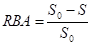(3)

In Eq. 3, S is the light scattering coefficient of the sample sheet and S0 is the total light scattering coefficient for completely unbonded fibers in a sheet. Because the RBA measurement can be done more quickly by using the light scattering method, it has been preferred over the nitrogen absorption method (Swanson and Steber 1959). However, it is not suitable for very thin paper sheets because the real value of S0 is smaller than the measured value for this material (Niskanen and Pajatora 2002). Furthermore, this method is unsuitable for measuring the fiber bonding in machine-made paper sheets.

In our work, the fiber characteristics (length, width, and coarseness) and strength properties of different poplar P-RC APMP fiber fractions were measured, and their Page bonding strength index (B), b, and RBA values were calculated based on a series of equations. Finally, the interfiber bonding abilities of different P-RC APMP fiber fractions were compared, which could be useful for evaluating the fiber quality of HYP pulps and further optimizing the process parameters in HYP production lines.

EXPERIMENTAL

Materials

A kind of poplar P-RC APMP pulp, collected from a paper mill in Shandong province in China, was classified by a Bauer-McNett fiber classifier (TMI 8901-05, USA) into different fiber fractions according to TAPPI standard method T233 cm-95 (1995), including R30, P30/R50, P50/R100, and P100/R200. Four fiber fractions were collected; R30 and P30/R50 acted as the long-fiber fractions, P50/R100 were the middle-fiber fraction, and P100/R200 were the short-fiber fractions.

Methods

Fiber characteristics

The fiber characteristics of each fraction, including average fiber length, average fiber width, coarseness, and other properties, were measured using a Fiber Tester (921 L&W; Sweden). For each measurement, there were about 10,000 fibers used.

Handsheet preparation

Handsheets with a basis weight of 60 g/m2 were made from each fiber fraction on a laboratory sheet former (Lab Tech; Canada) in accordance with TAPPI standard T205 sp-95 (1995). Tap water was used for the handsheet preparation, without whitewater circling. An automatic sheet press (No. 2571-1, KRK; Japan) was used for the wet pressing with varying gauge pressures of 0, 0.1, 0.2, 0.4 and 0.6 MPa. The pressing time was 5 min for one side, and another 2 min for the other side. After pressing, the handsheets were moved to an equilibrium room with a (50±2)% RH and (23±1)ºC for drying. In addition, eight handsheet samples were prepared for each case to ensure the accuracy of experimental data.

Measurement of physical properties

The light scattering coefficient was measured with an Elrepho Spectrophotometer (SE070 L&W; Sweden) in accordance with TAPPI standard T425 om-91 (1991). The tensile strength and the zero-span tensile strength were measured respectively by a tensile tester (L&W; Sweden) and a Z-span Tester (2400 PULMAC; USA) following TAPPI standard T220 sp-96 (1996). For each trial, eight parallel measurements were done, and finally the average could be obtained.

RESULTS AND DISCUSSION

Fiber Characteristics and Physical Properties of Different Fractions

The fiber characteristics of different poplar P-RC APMP fractions are summarized in Table 1. The values of mean fiber length and width reported were the weighted average and the coarseness given directly by the Fiber Tester. As P-RC APMP fibers had not been distorted and damaged, they can be considered to be cylindrical and the width of the fiber can be regarded as the diameter. Then, the mean fiber perimeters can be calculated.

Table 1. Fiber Characteristics of Different Poplar P-RC APMP Fiber Fractions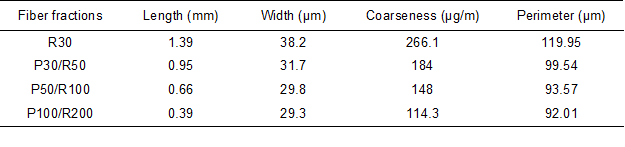As shown in Table 1, when the screen mesh increased from 30-mesh to 200-mesh, the fiber length, width, coarseness, and perimeter decreased by 71.9%, 23.3%, 57.0%, and 23.3%, respectively. Usually, shorter fiber fractions are subjected to more mechanical forces and become more fibrillated, resulting in a lower fiber length, width, and coarseness. With large changes in the fiber characteristics, the physical properties led to the assumption that different fractions must be diverse.

The physical properties of handsheets formed using different poplar P-RC APMP fiber fractions, which were made under a series of wet pressing pressures, are listed in Table 2.

Table 2. Physical Properties of Different Poplar P-RC APMP Fiber Fractions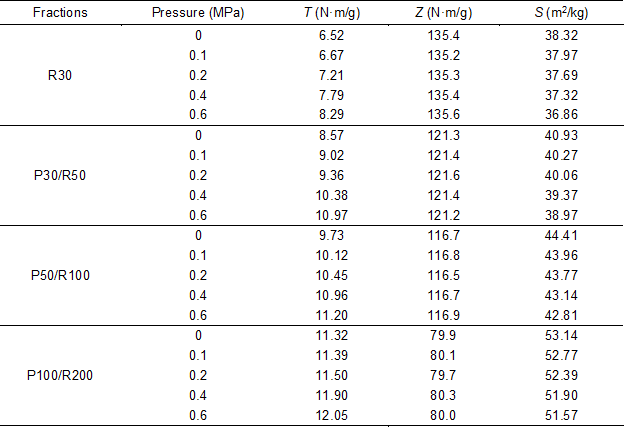It can be seen that for handsheets made from the same fiber fraction, with an increase in wet pressing pressures, the tensile index (T) had an obvious increase, while the light scattering coefficient (S) decreased. The zero-span tensile index (Z) had almost no change, which indicates that the wet press had no effect on the zero-span tensile index of handsheets with the same fiber fractions. For example, for the fraction of R30, with wet pressing pressures varying from 0 to 0.6 MPa, the tensile indices increased by 27.15% (from 6.52 N.m/g to 8.29 N.m/g) and the light scattering coefficient was reduced by 3.81% (from 38.32 m2/kg to 36.86 m2/kg). This is due to increasing bonded area between fibers with the increase of wet pressing. These results are in agreement with the conclusions from previous studies. (I’Anson and Karademir 2006). Similarly, as the wet pressing pressure of the R30, P30/R50, P50/R100, and P100/R200 fractions increased from 0 to 0.6 MPa, the percent increase in the tensile indices were 27.15%, 28.00%, 15.11%, and 6.45%, respectively, while the percent decreases in the light scattering coefficients were 3.81%, 4.79%, 3.60%, and 2.95%, respectively. As a result, it was concluded that, with an increase in screen mesh from 30-mesh to 200-mesh, the effect of wet pressing increased at first and then decreased. Furthermore, the wet pressing led to a great improvement in the fiber bonding between the long-fiber fractions and the middle-fiber fractions, but it caused a smaller improvement in the short-fiber fractions. The response order was as follows: P30/R50 > R30 > P50/R100 > P100/R200. With an increasing wet pressure, the extent of the increase of the tensile index from the fractions of R30 to P100/R200 decreased, while the extent of the increase of the light scattering coefficient was raised. The zero-span tensile index remained unchanged.

For practical application, the process parameters for P-RC APMP production can be optimized to improve the ratios of middle or short fiber fractions, and further to improve the interfiber bonding ability of HYP whole pulp. Also, the relatively strong wet pressing during the papermaking process can be made to increase the strength properties of HYP-containing paper sheets.

Calculation of RBA and b

By simplifying the variable changes for the Page Equation, a method for computation of relative bonded area (RBA) and shear bond strength per unit area (b) was introduced (Tao et al. 2005a, b; 2006).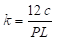(4)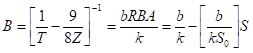(5)

In these equations, k (g/m3) is a parameter related to the fiber characteristics and is the page bonding strength index (Nm/g).

The pressing had no effect on the shear bonding strength per unit area (b) or the fiber characteristics (k) (I’Anson and Karademir 2006). Using the fiber characteristics data listed in Table 1, the parameter kfor different P-RC APMP fiber fractions could be calculated using Eq. 4. The tensile index (T) and the zero-span tensile index (Z) listed in Table 2 were used in Eq. 4, and the parameter B was obtained for different fiber fractions under different wet pressing pressures. The linear relationship between the light scattering coefficient (S) and the bonding strength index (B) were plotted in Fig. 1 according to Eq. 5.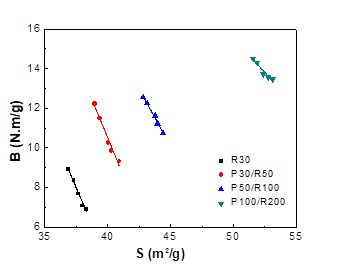Fig. 1. Linear relationship between the Page bonding strength index (B) and the light scattering coefficient (S)

The horizontal axis intercepts, b/k in Eq. 4, and the slopes of the lines, –b/(kS0) in Eq. 5, were calculated according to Fig. 1 to obtain band S0. The fitting functions of the linear relationship between S and B, and the results of k and S0 for different fiber fractions, are listed in Table 3.

Table 3. The kb, and S0 of Fibers and Handsheets of Different Fiber Fractions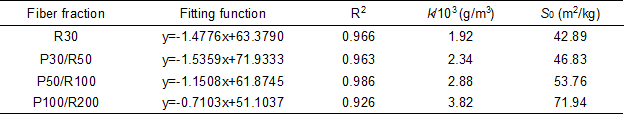As the screen mesh increased from 30-mesh to 200-mesh, k and S0increased; that is, as the fiber morphology (fiber length, fiber width, and fiber coarseness, listed in Table 1) decreased, Sincreased dramatically.

A histogram of the shear bond strength per unit area (b) of different poplar P-RC APMP fiber fractions is shown in Fig. 2. The shear bond strength per unit area (b) increased with increasing fiber size. This may be the result of the P-RC APMP production process. The shorter fiber fractions were subjected to a more severe mechanical shearing action during the refining process, which removed more middle lamella than were removed in longer fiber fractions. Because of this difference, the shorter fibers may have less surface lignin coverage and larger specific surface area, exposing more hydroxyl groups at the fiber surface. These shorter fibers enhanced the hydrogen bonding between fibers and resulted in relatively large shear bond strength per unit area (b).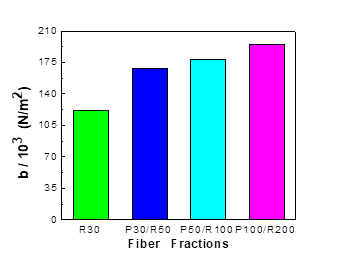Fig. 2. Shear bond strength per unit area (b) of different poplar P-RC APMP fiber fractions

The RBA values of handsheets made from different poplar fiber fractions under different wet pressing pressures were calculated based on Eq. 2; the data are shown in Table 4. A histogram of the RBA of different poplar P-RC APMP fiber fractions with different wet pressing pressures is shown in Fig. 3.

Table 4. RBA of Handsheets Made from Different Poplar P-RC APMP Fiber Fractions under Different Pressures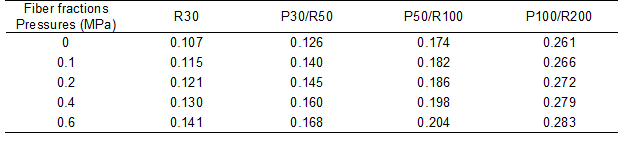As shown in Fig. 3, at a fixed wet pressing pressure, the RBA of the handsheets increased dramatically with increasing fiber size, especially for the P100/R200 fraction. The RBA of handsheets made from these fibers ranged from approximately 0.26 to 0.28. Also, the wet pressing had a positive effect on the RBA. When the wet pressing pressure increased, the fiber matrix in the paper sheets’ structure was squashed, and more bonded areas on the fiber surface could be obtained. Smaller fiber fractions have a larger specific surface area and more opportunities for bonding with each other, generating a higher RBA.

As an important parameter of fiber bonding, B was positively proportional to the values of b and RBA of handsheets and negatively proportional to the k of fiber fractions. The B values exhibited in Fig. 1 increased for different fiber fractions in correspondence with the increase in kb, and RBA. The B value of the handsheets increased with increasing kb, and RBA of the fibers. Therefore, it is important to adjust the ratios of P-RC APMP fiber fractions to obtain the optimal combination of kb, and RBA that can improve the physical strength of HYP-containing paper sheets.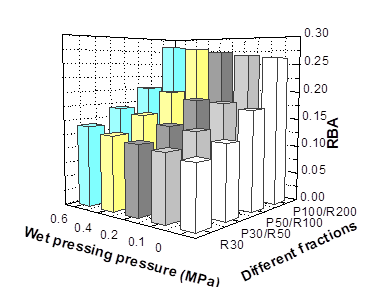Fig. 3. RBA of different poplar P-RC APMP fiber fractions with different wet pressing pressure

CONCLUSIONS

1. By simplifying the variable changes for the Page Equation, RBA and b were estimated using the fiber characteristics and physical properties of handsheets made from different poplar P-RC APMP fiber fractions under different wet pressing.
2. The handsheets made from shorter fiber fractions had higher BRBA, and b. The wet pressing had a positive effect on the interfiber bonding, leading to a great improvement for the long- and middle-fiber fractions, but a small improvement for the short-fiber fraction. Also, relatively strong wet pressing during papermaking process can increase the strength properties of HYP-containing paper sheets.
3. In practice, the process parameters would be optimized during HYP production and application to improve the interfiber bonding ability, if the process conditions and production costs allow.

ACKNOWLEDGMENTS

We acknowledge the financial support of the National Natural Science Foundation of China (Grant No. 31370577), and the Foundation for Tianjin Municipal Science and Technology Support Key Projects (Grant No. 12ZCZDGX01100).

REFERENCES CITED

I’Anson, S. J., Karademir, A. and Sampson, W. W. (2006). “Specific contact area and the tensile strength of paper,” Appita J. 59(4), 297-301.

Lei, M., Zhang, H., Li, J., and Duan, J. (2013). “Characteristics of poplar preconditioning followed by refining chemical treatment alkaline peroxide mechanical pulp fiber fractions and their effects on formation and properties of high-yield pulp containing paper,” Ind. Eng. Chem. Res. 52(11), 4083-4088.

Niskanen, K., and Pajatora, H. (2002). “Statistical geometry of paper cross-sections,” J. Pulp Paper Sci. 28(7), 228-233.

Page, D. H. (1969). “A theory for the tensile strength of paper,”TAPPI J. 52(4), 674.

Skowronski, J., and Bichard, W. (1987). “Fiber to fiber bonds in paper. Part I: Measurement of bond strength and specific bond strength,” J. Pulp Paper Sci. 13(5), 165-169.

Swanson, J. W., and Steber, A. J. (1959). “Fiber surface area and bonded area,” TAPPI J. 42(1), 986-994.

Tao, J., Liu, H., Yan, D., Ge, H., and Kou, S. (2006). “A new method for determining fiber-fiber shear bond strength,” Trans. China Pulp & Pap. 21(4), 74-80.

Tao, J., Yan, D., Liu, H., Ge, H., Shen, W., Xu, Y., and Chen, X. (2005a). “A study of the relation between the relative bonded area and the fiber flexibility (I),” J. Shaanxi Univ. Sci. Technol. 23(5), 6-11.

Tao, J., Yan, D., Liu, H., Ge, H., Shen, W., Xu, Y., and Chen, X. (2005b). “A study of the relation between the relative bonded area and the fiber flexibility (II),” J. Shaanxi Univ. Sci. Technol. 23(6), 1-4.

TAPPI. T 205 sp-95. (1995). “Forming handsheets for physical tests of pulp”.

TAPPI. T 220 sp-96. (1996). “Physical testing of pulp handsheets”.

TAPPI. T 233 cm-95. (1995). “Fiber length of pulp by classification”.

TAPPI. T 425 om-91. (1991). “Opacity of paper (15°/diffuse, illuminant A, 89% reflectance backing and paper backing)”.

Xu, E. C. (1999). “A new concept in alkaline peroxide refiner mechanical pulping,” Proceedings of International Mechanical Pulping Conference, Houston, TX, pp. 5A-3.

Article submitted: April 3, 2014; Peer review completed: June 24, 2014; Revised version received and accepted: August 10, 2014; Published: August 18, 2014.